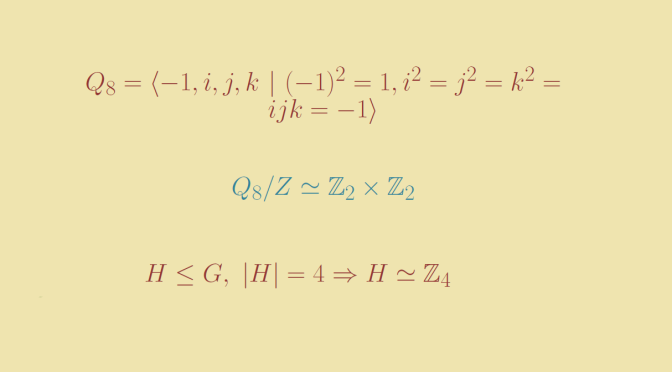Is the quotient group of a finite group always isomorphic to a subgroup?

Given a normal subgroup $$H$$ of a finite group $$G$$, is $$G/H$$ always isomorphic to a subgroup $$K \le G$$?

The case of an abelian group

According to the fundamental theorem of finite abelian groups, every finite abelian group $$G$$ can be expressed as the direct sum of cyclic subgroups of prime-power order: $G \simeq \bigoplus_{i=1}^u \mathbb{Z}_{p_i^{\alpha_i}}$ where $$p_1, \dots , p_u$$ are primes and $$\alpha_1, \dots , \alpha_u$$ non zero integers.

If $$H \le G$$ we have $H \simeq \bigoplus_{i=1}^u \mathbb{Z}_{p_i^{\beta_i}}$ with $$0 \le \beta_1 \le \alpha_1, \dots, 0 \le \beta_u \le \alpha_u$$. Then $G/H \simeq \bigoplus_{i=1}^u \mathbb{Z}_{p_i^{\alpha_i-\beta_i}}$ which is a subgroup of $$G$$.

If $$G$$ is not abelian, then $$G/H$$ might not be isomorphic to a subgroup of $$G$$.

The case of the quaternion group $$Q_8$$

Consider the quaternion group $Q_8 =\langle -1,i,j,k \ | \ (-1)^2=1,i^2=j^2=k^2=ijk=-1\rangle$
The center of $$Q_8$$ is $$Z=\{-1,1\}$$ as we have the relations:$\left\{ \begin{array}{ccc} ij=k & , & ji=-k \\ jk=i & , & kj=-i \\ ki=j & , & ij=-i \\ \end{array} \right.$
$$Q_8/Z$$ has order four and all elements of $$Q_8/Z$$ have order two as for $$x \in Q_8$$, $$x^2 \in \{-1,1\}$$. On the other hand the order four subgroups of $$Q_8$$ are $$\langle i \rangle, \langle j \rangle, \langle k \rangle$$ which are cyclic and isomorphic to $$\mathbb Z_4$$.

Finally $$Q_8/Z$$ cannot be isomorphic to a subgroup of $$G$$.